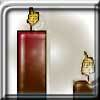#### You may also like### Burning Down

One night two candles were lit. Can you work out how long each candle was originally?### Percentage Unchanged

If the base of a rectangle is increased by 10% and the area is unchanged, by what percentage is the width decreased by ?### Digit Sum

What is the sum of all the digits in all the integers from one to one million?

# Adding a Square to a Cube

##### Age 14 to 16 ShortChallenge Level

Answer: $n$ could be $3, 8, 15, 24, 35, 48, 63, 80$ or $99$

$n^2+n^3=n^2(1+n)$

$(ab)^2=ab\times ab=a\times a\times b\times b=a^2\times b^2$,
So if $(1+n)$ is square then $n^2(1+n)$ will be square

For example, if $1+n = 2^2 = 4$, then $n=3$, and $n^2(1+n)=3^2\times2^2=\left(3\times2\right)^2=6^2$. So $n^2+n^3$ is a square number (you can also check that $n^2+n^3=36$.)

$1+n$ could be $4, 9, 16, 25, 36, 49, 64, 81$ or $100$
$n$   could be $3, 8, 15, 24, 35, 48, 63, 80$ or $99$

Those are 9 possible values of $n$, so they must be the 9 values we were looking for.

You can find more short problems, arranged by curriculum topic, in our short problems collection.